Michael Fowler

University of Virginia

We shall now turn to another puzzle confronting physicists at the turn of the century -- just how heated bodies radiate. There was a general understanding of the mechanism involved -- heat was known to cause the molecules and atoms of a solid to vibrate, and it was known that the molecules and atoms were themselves complicated patterns of electrical charges. From the experiments of Hertz and others Maxwell's predictions that oscillating charges emitted electromagnetic radiation had been confirmed, at least for simple antennas. It was known from Maxwell's equations that this radiation traveled at the speed of light and from this it was realized that light itself, and the closely related infrared heat radiation, were actually electromagnetic waves. The picture, then, was that when a body was heated, the consequent vibrations on a molecular and atomic scale included some oscillating charges. If one assumed that Maxwell's theory of electromagnetic radiation, which worked well in the macroscopic world, was also valid at the microscopic scale (tenths of nanometers), then these oscillating charges would radiate, presumably giving off heat and light.

What is meant by the phrase "black body" radiation? The point is that radiation from heated bodies depends to some extent on the body heated. Let us back up momentarily and consider how differently different materials absorb radiation. Some, like glass, seem to absorb light hardly at all-the light goes through. For a shiny metallic surface, the light isn't absorbed either, it gets reflected. For a black material like soot, light and heat are almost completely absorbed, and the material gets warm. How can we understand these different behaviors in terms of light as an electromagnetic wave interacting with charges in the material, causing them to oscillate and absorb energy from the radiation? In the case of glass, evidently this doesn't happen, at least not much. A full understanding of how this works needs quantum mechanics, but the general idea is as follows. There are charges-electrons-in glass that are able to oscillate in response to an applied external oscillating electric field, but these charges are tightly bound to atoms, and only oscillate at certain frequencies. It happens that for ordinary glass none of these frequencies correspond to those of visible light, so there is no resonance with a light wave, and hence little energy absorbed. Glass is opaque at some frequencies outside the visible range (in general, both in the infrared and the ultraviolet). These are the frequencies at which the electrical charge distribution in the atoms or bonds can naturally oscillate.

A piece of metal has electrons free to move through the entire solid. This is why metals can conduct electricity. It is also why they are shiny. These unattached electrons oscillate together with large amplitude in response to the electrical field of an incoming light wave. They themselves then radiate electromagnetically, just like a current in an antenna. This radiation from the oscillating electrons is the reflected light. In this situation, little of the incoming radiant energy is absorbed, it is just reradiated, that is, reflected.

Soot, like a metal, will conduct an electric current, although not nearly so well. There are unattached electrons, which can move through the whole solid, but they keep bumping into things-they have a short mean free path. When they bump, they cause vibration, like a pinball machine, so they give up energy into heat. Although the electrons in soot have a short mean free path compared to that in a good metal, they move very freely compared with electrons in atoms, so they can accelerate and pick up energy from the electric field in the light wave. They are therefore effective intermediaries in transferring energy from the light wave into heat.

### Absorption and Emission

Heated bodies radiate by processes just like the absorption described above operating in reverse. Thus, for soot heat causes the lattice to vibrate more vigorously, giving energy to the electrons (imagine them as balls in a pinball machine with strongly vibrating barriers, etc.) and since the electrons are charged they radiate away excess kinetic energy. On the other hand, the electrons in a metal have very long mean free paths, the lattice vibrations affect them much less, so they are less effective in radiating away heat. It is evident from considerations like this that good absorbers of radiation are also good emitters.

At sufficiently high temperatures, all bodies become good radiators. Items heated until they glow in a fire look much more similar than they do at room temperature. For a metal, this can be understood in terms of a shortening of the mean free path by the stronger vibrations of the lattice interfering with the electron's passage.

### Observing the Black Body Spectrum

Any body at any temperature above absolute zero will radiate to some extent, but the intensity and frequency distribution of the radiation depends on the detailed structure of the body. To make any progress in understanding radiation, we must specify the details of the body radiating. The simplest possible model is to consider a body which is a perfect absorber, and therefore the best possible emitter. For obvious reasons, this is called a "black body".

A good model absorber is a small hole in the side of a box. Radiation impinging on the hole from outside enters the box and is absorbed as it is scattered around inside, so only a tiny fraction is re-emitted.

We shall, therefore, take a cubical box (an oven, in other words) with a small hole in the side as our model to analyze emission of radiation. Fortunately, this is a system which can also be investigated experimentally fairly easily. A beam of radiation from the hole in the oven is passed through a diffraction grating and projected on to a screen, where it is separated out by wavelength (or equivalently frequency). A detector is moved up and down along the screen to find how much radiant energy is being emitted in each frequency range, say the energy between frequency f and f + df is RT(f)df.

We can then plot RT(f) against f for a given temperature T. It is found experimentally that for small f, RT(f) is proportional to f2, a parabolic shape, but for increasing f it falls below the parabola, peaks at fmax, then falls quite rapidly to zero as f increases beyond fmax.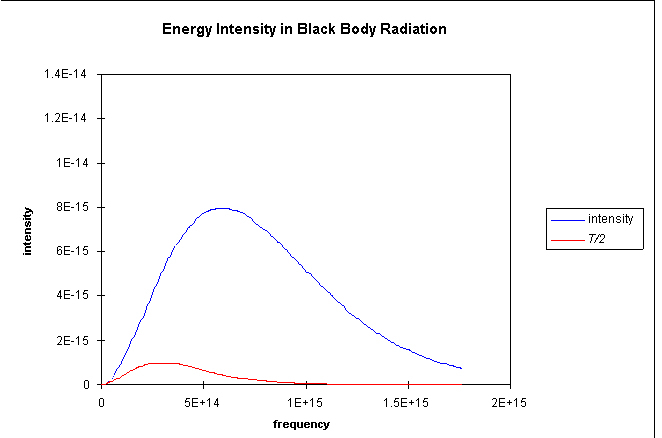Suppose we now double the absolute temperature - how does that affect RT(f)?

For those low frequencies where RT(f) was parabolic, doubling the temperature doubles the intensity of the radiation. However, it is found that at 2T the curve follows the (doubled) parabolic path much further - in fact, twice as far: fmax(2T) = 2fmax(T).

The curve R2T(f), then, reaches eight times the height of RT(f). It also spreads over twice the lateral extent, so the area under the curve, corresponding to the total energy radiated, increases sixteenfold on doubling the temperature. This is Stefan's Law of Radiation: the power P radiated from one square meter of black surface at temperature T: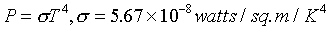Note that the graph above plots the energy intensity in the oven. This energy is being pumped out of the opening at speed c. When allowance is made for the fact that an opening of unit area is effectively less than unit area for radiation coming in at an angle, and half the energy is in waves moving in directions away from the whole, the actual rate of emission of energy through a hole of unit area is equal to (1/4)c times the energy intensity inside.

The upward shift in fmax with T is something everyone is familiar with - when an iron is heated in a fire, the first visible radiation is deep red - the lowest frequency visible light. Further increase in T causes the color to change to orange then yellow, and white at a very high temperature, signifying that all the visible frequencies are being emitted roughly equally.

The change in fmax is linear in the absolute temperature;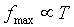This is called Wein's Displacement Law.

For example, the sun's surface temperature is 5700K, and at that temperature much of the energy is radiated as visible light. This is no accident - evolution has adapted us to see most efficiently in the light most readily available.

This shift in the frequency at which radiant power is a maximum is very important for harnessing solar energy, such as in a greenhouse. We need glass which will allow the solar radiation in, but not let the heat radiation out. This is feasible because the two radiations are in very different frequency ranges - 5700K and, say, 300K - and there are materials transparent to light but opaque to infrared radiation. This is only possible because fmax varies with temperature.

### Understanding the Black Body Spectrum: the Analogy with a Gas

Experimental investigations of the radiation intensity as a function of temperature and frequency, RT(f) above, give well-defined reproducible results, not very sensitive to the material of the oven, etc. For a small hole, the radiation emitted will be a representative sample of the radiation bouncing around inside the box.

In fact, the general shape of the function RT(f) was very reminiscent of the energy distribution in a gas in thermal equilibrium, as analyzed by Maxwell and Boltzmann. In contrast to the molecules in a gas, the different wavelengths of radiation do not collide with each other in the middle of the oven. Nevertheless, energy can shift from one mode to the other through the intermediary of the oscillators in the wall - which is to say, just the atoms and molecules the wall is made of. An electromagnetic wave in the oven can set a charge oscillating in the wall, energy can be transferred to other charges in the wall, which will in general oscillate and radiate at different frequencies. Thus the different frequencies of radiation inside the oven will come to thermal equilibrium.

### Counting the Degrees of Freedom

To make any quantitative progress in analyzing the radiation, we must have a clear picture of the degrees of freedom of this system - how many oscillators have frequencies in a given energy range? These oscillations are standing electromagnetic waves. The waves are contained in the oven, so the electric field intensity drops rapidly to zero on approaching and going into the walls, because inside the walls the electric energy will be rapidly dissipated by currents or polarization. In fact, then, the boundary condition at the walls is much like that for waves on a string fixed at both ends, where the wave amplitude goes to zero at the ends.

As a warm up exercise, consider the frequency distribution function of the possible different modes of vibration (that is, the different degrees of freedom) of a string stretched between two points a distance a apart. Possible values of the wavelength are: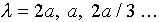so the frequency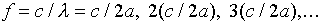The allowed frequencies are thus equally spaced c/2a apart. We define the spectral density by stating that the number of modes between f and f + delta_f is N(f)delta_f for delta_f small compared with the range of frequencies in the system, but large compared with the spacing between successive allowed frequencies. Evidently, in this simple one-dimensional example N(f) is a constant equal to 2a/c.

The amplitude of oscillation as a function of time has the form: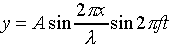This is more conveniently written: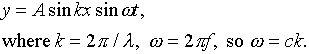Here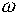is the radial frequency - how many radians per second, rather than cycles per second, the time dependent component of the wave moves through. Analogously, k, called the wave number, counts the radians of spatial change in unit length (such as one meter).

We are now ready to tackle the more complex problem of three-dimensional standing electromagnetic waves in a cubical oven. The first guess, just generalizing the expression above, would be: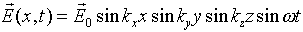This will satisfy the wave equation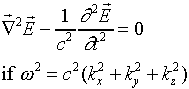Also the electric field will be zero at all the walls if we choose the k's appropriately, that is, so that sinkxa = 0, etc.

However, this wave is not quite correct, because since there is no electric charge in the oven away from the walls, the divergence of the electric field must be identically zero away from the walls, a condition this wave does not satisfy. The divergence is: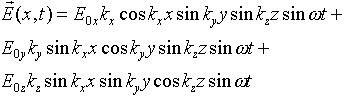and, for example, taking any x for which sinkxx = 0, the second and third terms above are identically zero, but the first term certainly isn't.

This problem can be fixed by thinking more carefully about the boundary conditions at the walls. It is true that any component of the electric field parallel to the wall will be attenuated rapidly by currents or polarization in the wall. However, there could be an electric field perpendicular to the wall, because there could be surface charge on the wall. This means a possible solution is: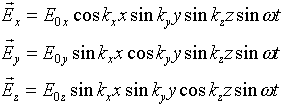These components are deliberately chosen to give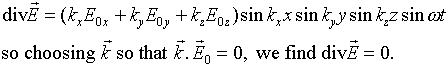This is telling us something we should have realized from the beginning - in the standing electromagnetic wave in the cavity, just as in a propagating wave, the electric field is perpendicular to the direction in which the wave is moving. In other words, it is a transverse wave, and in fact there are two independent polarizations, which we must remember to count when we find the total number of degrees of freedom.

It remains to find the allowed frequencies of vibration - the normal modes - of the electromagnetic radiation in the oven. The first step is to find the allowed values of the wave numbers kx, ky and kz. These are fixed by the boundary conditions sinkxa = 0, etc., so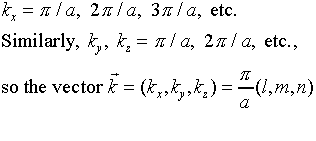where( l, m, n) are positive integers, like (1, 1, 1) or (5, 13, 4) - in other words, each possible standing electromagnetic wave in the oven corresponds to a point in the (kx, ky, kz) space labeled by three positive integers. These are the intersection points of a cubic lattice in the space kx > 0, ky > 0, kz > 0.

Each such point can be associated with the little lattice cube of volume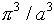for which the point in question is the furthest corner of the little cube from the k-space origin. These little cubes stack together to fill all that part of k-space having kx > 0, ky > 0 and kz > 0.

The frequency of vibration of the wave having wave numbers (kx, ky, kz) isRecall that the experimental measurement of black body radiation from the oven detects the intensity of radiation in a given frequency range. It does not tell us the wave numbers of the radiating modes! Hence, to compare with these experimental results, we must choose a small frequency range and find how many possible sets of wave numbers correspond to modes of vibration having frequencies within that range.

Notice that a fixed value of the frequency corresponds to a spherical surface in k-space:Therefore, the number of possible modes of radiation in the oven having frequencies in the range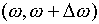is equal to the number of lattice-point wave number values (kx, ky, kz) between two spherical surfaces centered at the origin and having radii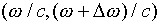, and only counting in the octant corresponding to kx > 0, ky > 0 and kz > 0.

Now, as we argues above, each lattice point can be associated with a small cube of volume. Assuming we choose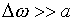, there will be many of these small cubes between the spherical surfaces, and the total number of lattice points between the spheres in the positive octant will be just the volume of the space between the spheres divided by the volume of one of these cubes.

So the number of possible modes of radiation in the oven having frequencies in the rangeis: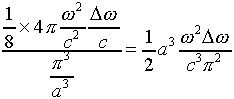Putting this in terms of the frequency f in cycles per second,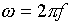, and inserting an extra factor of two for the two independent polarizations of the wave (discussed above) we find the distribution function for modes in frequency is given by: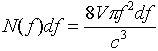where V = a3 is the volume of the oven.

### What about Equipartition of Energy?

The point of the rather tedious calculation above, finding exactly how many different modes of vibration, or degrees of freedom, there are in the ovenfull of radiation, was to construct an argument parallel to that applied successfully to understand the properties of gases. The first conclusion would be that there is kT of energy, on average in each wave mode (not ½kT, because the standing wave is like an oscillator with both kinetic and potential energy).

For low frequencies, this is exactly what is observed. The amount of radiation shining out of the oven at low frequencies is correctly predicted by counting modes, as above, and allocating kT of energy to each mode.

### The Ultraviolet Catastrophe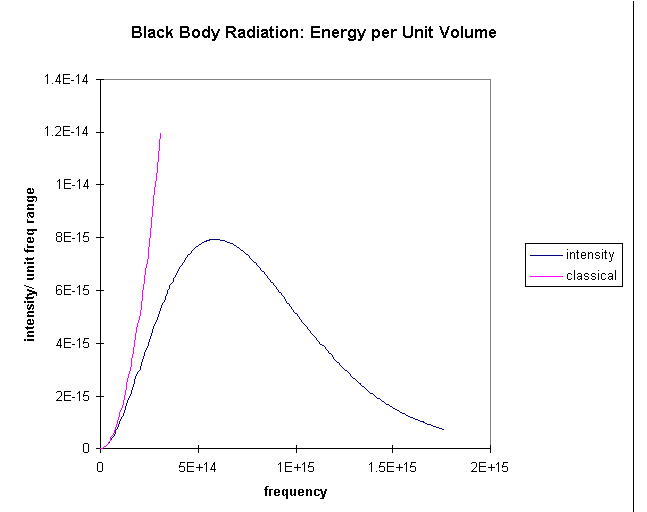The problem is that as we go to higher frequencies, there are more and more possible degrees of freedom. The oven should be radiating huge amounts of energy in the blue and ultraviolet. But it isn't. For this system, the equipartition of energy isn't working!

The graph shows the prediction of radiation intensity from the classical equipartition compared with the experimental result. It is evident that the higher frequency modes are not getting their "fair share" kT of energy, somehow they are getting frozen out, evidently those at really high frequencies are getting almost no share in the energy at all.

It is important at this point to go back to the curves earlier in this lecture for two different temperatures, and ask the question: how is the sharing of energy among the modes affected by raising the temperature? We provide below a magnified version of the low frequency parts of the curves for T/2 and T, and show for each case the classical prediction based on the mode counting in the preceding section. Look at the curves and think about this before reading on!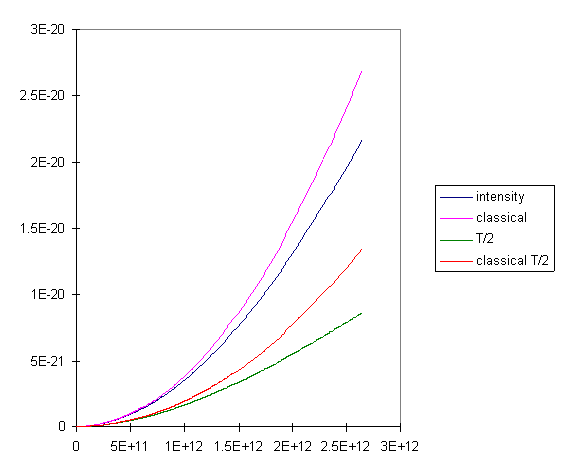It is clear that for the lowest frequencies, doubling the temperature does indeed double the intensity of the radiation, exactly what we expect if the radiation is proportional to kT, the energy in each mode. (T = 300K here, by the way.) We can also see, though, that at the higher temperature, the observed radiation intensity stays closer to the classical prediction as we go to higher frequencies. This means the higher modes are less locked out of the energy sharing when we go to higher temperatures. For any given temperature, though, if we go to high enough frequencies, the intensity drops exponentially with further increase in frequency.

### Planck's Suggestion

Planck suggested that this lack of equipartition could be understood if the oscillators in the walls of the oven were somehow constrained so that they could not continuously emit radiation, as Maxwell's equations would predict, but could only emit it in chunks - called quanta - of a definite magnitude. For higher frequencies, he suggested, the radiation had to be emitted in bigger chunks. This would explain the lack of radiation in the oven at these higher frequencies - assuming the oscillators in the walls had on average energy kT, it would be very unlikely that one oscillating charge would by random excitation have an energy of, say, 5kT, so if its frequency of oscillation were high enough, it would almost never have enough energy to emit one quantum, so by Planck's theory, it wouldn't emit any radiation at all. On the other hand, if the temperature were increased fivefold, it would often have enough energy to emit a quantum.

Given, then, that bigger chunks of energy are emitted at the higher frequencies, just how does the size of a chunk depend on the frequency of the radiation? The clue is given by the Wein displacement formula,. Now, fmax is the frequency at which the curve turns over and begins to descend, moving far from the classically predicted parabola, which of course continues upwards. Thus, doubling the temperature doubles the frequency range over which the curve approximates the classically predicted parabola. (If we stiffen the requirements for approximating the classical curve, for example by requiring it to be within 5%, say, then we can see from the graph of low energy modes above that doubling the temperature still doubles the range over which the curves remain close.)

The bottom line is that doubling the temperature apparently doubles the frequency range in which oscillators get approximately their full share kT of energy. But doubling the temperature doubles the size of a chunk of energy that can be easily moved. This leads to the conclusion that if two oscillators have frequencies f and 2f, the one at 2f must emit energy in quanta just twice the size of those emitted by the one at f. The size of the energy quantum therefore depends linearly on the frequency,

E = hf

where h is Planck's constant.

The way this evolved historically was that at first the higher frequency part of the black body radiation curve was mapped out by experimentalists in Berlin, and Wien showed that it was well approximated by the formulawith A a constant. This was very reminiscent of the Maxwell distribution for gas molecules, but with f3 in place of v2. It soon became evident, though, that the formula was not accurate at low frequencies, where in fact it appeared that the distribution went as f2, as discussed above. Planck then wrote down a formula having the right behavior for both high and low frequencies: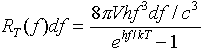He did not at first have any theoretical justification for this formula, but it was a very accurate fit to some very precise experiments.

It is easy to see how Wien's Displacement Law follows from this formula --- the maximum radiation per unit frequency range is at the frequency f for which the function f3/(ehf/kT-1) is a maximum. Solving numerically gives hfmax=2.82kT. (It is perhaps worth mentioning that this is the frequency range at which the radiation per unit frequency range is a maximum. In some older books, Wien's law is written in terms of wavelengths, and gives the wavelength at which the radiation per unit wavelength range is maximum. Because the wavelength and frequency are not linearly related, but in fact inversely related, these two maxima are not at the same frequency!)

### Justifying Planck's Formula

Factoring out the number of modes of oscillation in the frequency range df, we see that Planck's formula tells us that the average energy per mode is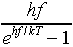For low frequencies, hf << kT, this correctly gives kT per mode. The formula follows if we assume the oscillator can only have a whole number of quanta of energy, that is to say, its energy must be one of: 0, hf, 2hf, 3hf, … If we further assume that the relative probability of it having energy E is e-E/kT, then its relative probabilities of having energy 0, hf, 2hf, … are in the ratio 1: e-hf/kT : e-2hf/kT, etc. The actual probabilities are given by dividing these relative probabilities by the sum of all of them They clearly are the terms of a geometric series, so their sum is just 1/(1 - e-hf/kT). So, to find the average energy in the oscillator, we take the possible energies 0, hf, 2hf, 3hf, … and weight each of them with their probability of occurring, that is, we must find 0.1 + hf. e-hf/kT +2hf. e-2hf/kT + … , and divide the sum by 1/(1 - e-hf/kT). This is left as an exercise for the reader. Planck himself gave a more complete and elegant discussion, applying Boltzmann's ideas about entropy to simple harmonic oscillators having only certain energies allowed. In fact, he effectively derived the Boltzmann distribution for this system.